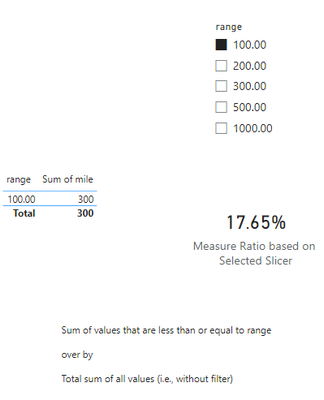cancel
Showing results for
Did you mean:New Member

## calculate ratio when numerator changes by applying slicer and denominator is fixed

I have a dataset with two columns: range and mile. there is an slicer on the range which shows max range. I want to calculate the ratio of mile/total mile, for example when max range is 300 the ratio equals summation of miles for rows with range<=300 (600) over total mile (1700) which is 0.35. I would appreciate if you help me to do this.

 range mile 300.00 200 200.00 100 500.00 500 100.00 300 1000.00 600 1700 range<=300 ratio=0.352941 range<=100 ratio=0.176471
1 ACCEPTED SOLUTIONSuper User

Try this: Tune to your needs

1) Table Name used as 'Q2 Table Ratio Slicer' and Slicer Name used as 'Slicer Range' (You can see the slicer name in view menu, show panes ... Selection, will get you the slicer name)

2) Measure used as

``````Measure Ratio based on Selected Slicer =
var _allTotal = CALCULATE(sum('Q2 Table Ratio Slicer'[mile]), all('Q2 Table Ratio Slicer'))
var _selSlicerMax = CALCULATE( MAX( 'Q2 Table Ratio Slicer'[range]), ALLSELECTED('Q2 Table Ratio Slicer'))
var _allValuesInRange = CALCULATE(sum('Q2 Table Ratio Slicer'[mile]),
filter(all('Q2 Table Ratio Slicer'), 'Q2 Table Ratio Slicer'[range] <= _selSlicerMax)
)

RETURN Divide(_allValuesInRange, _allTotal, blank())``````

Formatted the measure as %

Output 1: <No selection>Output 2: Selected: 300.00Output 3: Selected: 100.00Hope this helps!

2 REPLIES 2New Member

Thank you so much sevenhills.Super User

Try this: Tune to your needs

1) Table Name used as 'Q2 Table Ratio Slicer' and Slicer Name used as 'Slicer Range' (You can see the slicer name in view menu, show panes ... Selection, will get you the slicer name)

2) Measure used as

``````Measure Ratio based on Selected Slicer =
var _allTotal = CALCULATE(sum('Q2 Table Ratio Slicer'[mile]), all('Q2 Table Ratio Slicer'))
var _selSlicerMax = CALCULATE( MAX( 'Q2 Table Ratio Slicer'[range]), ALLSELECTED('Q2 Table Ratio Slicer'))
var _allValuesInRange = CALCULATE(sum('Q2 Table Ratio Slicer'[mile]),
filter(all('Q2 Table Ratio Slicer'), 'Q2 Table Ratio Slicer'[range] <= _selSlicerMax)
)

RETURN Divide(_allValuesInRange, _allTotal, blank())``````

Formatted the measure as %

Output 1: <No selection>Output 2: Selected: 300.00Output 3: Selected: 100.00Hope this helps!Announcements#### Exclusive opportunity for Women!

Join us for a free, hands-on Microsoft workshop led by women trainers for women where you will learn how to build a Dashboard in a Day!#### Power Platform Conference-Power BI and Fabric Sessions

Join us Oct 1 - 6 in Las Vegas for the Microsoft Power Platform Conference.Top Solution Authors
Top Kudoed Authors
Users online (1,940)